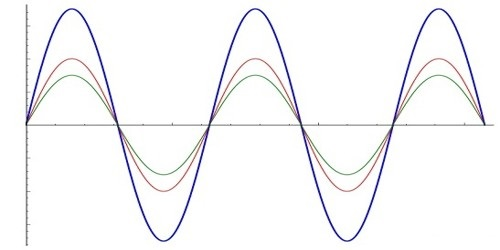# Conditions for Interference of Light

If two light-waves having same frequencies and amplitudes emitted from two coherent sources pass through the same point in a medium, the point becomes very bright when the two waves superpose at that point with the same phase and become dark if the two waves superpose with opposite phase. This phenomenon of variation of intensity of light created due to the superposition of light-waves is called interference.

Conditions for interference: The following conditions are needed to be fulfilled for interference-

(1) The two sources of light should be coherent sources.

(2) The two sources should be very fine and small.

(3) The two sources should be very close to each other.

(4) The amplitude of the two waves should be equal or nearly equal.

(5) For alternate bright and dark points, the path difference between the waves should be even multiples and odd multiples respectively of half wavelength.

If the above conditions are fulfilled, then interference will be observed.

Characteristics of Interference

1. Interference is produced by light waves from two coherent sources superposed at a point in a medium.
2. Normally the widths of the interference fringes are equal. Sometimes, they are unequal as well.
3. Distances between the bright and dark band are equal.
4. No light is found in interference fringes. They are always dark
5. The intensity of all the bright fringes is equal.